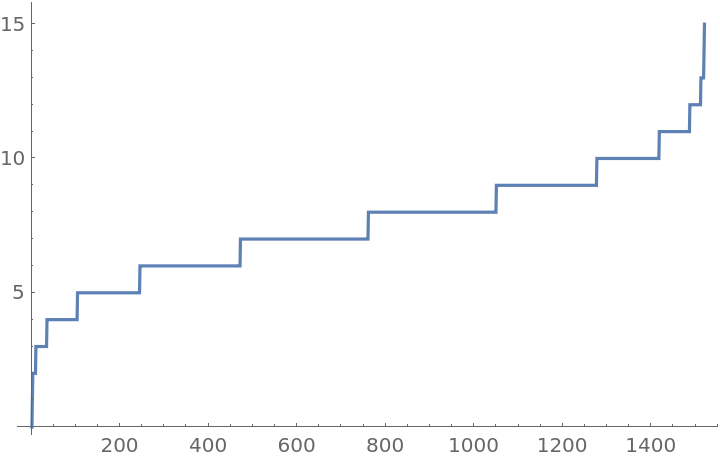#Function Repository Resource:

# SelectSubsets

Generate subsets that satisfy a certain criterion

Contributed by: Sander Huisman
 ResourceFunction["SelectSubsets"][list,crit] gives a list of all possible subsets of list that satisfy the criterion crit. ResourceFunction["SelectSubsets"][list,n,crit] gives all subsets containing at most n elements that satisfy crit. ResourceFunction["SelectSubsets"][list,{n},crit] gives all subsets containing exactly n elements that satisfy crit. ResourceFunction["SelectSubsets"][list,{nmin,nmax},crit] gives all subsets containing between nmin and nmax elements that satisfy crit. ResourceFunction["SelectSubsets"][list,nspec,crit,s] limits the results to the first s subsets.

## Details and Options

ResourceFunction["SelectSubsets"] orders subsets in the same way as Subsets. If the elements of list are in the order returned by Sort, then the complete result from SelectSubsets[list,crit] will also be in this order.
The setting All for nspec gives the subsets for the lengths 0 to Length[list].
ResourceFunction["SelectSubsets"][list,{nmin,nmax,dn},crit] gives subsets containing nmin, nmin+dn, … elements.

## Examples

### Basic Examples

Select subsets from {1,2,3,4,5} that add up to 10:

 In:=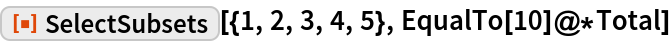Out=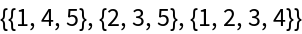Select subsets of length 2 to 4 that sum up to a prime:

 In:=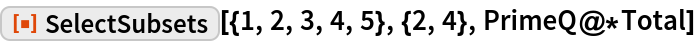Out=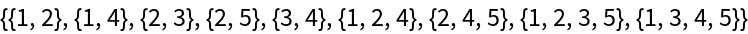### Scope

Select all subsets of length 2 that add up to 6:

 In:=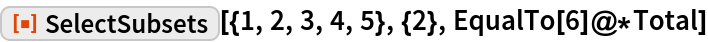Out=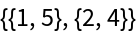Select all subsets that add up to 0:

 In:=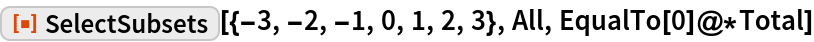Out=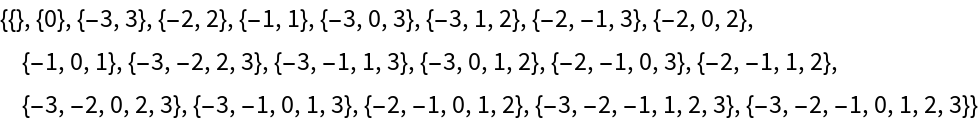Select all subsets of odd length that add up to a prime:

 In:=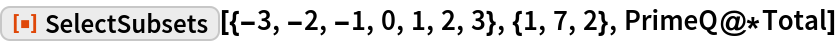Out=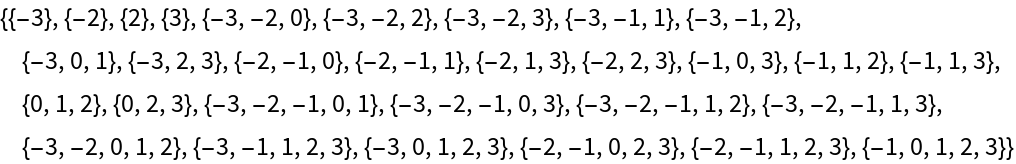Select the first 8 subsets that add up to a prime:

 In:=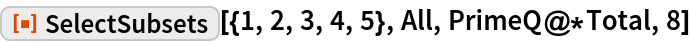Out=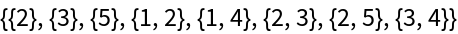### Applications

Find subsets that add up to 25:

 In:=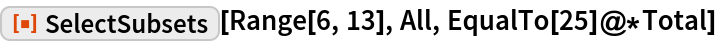Out=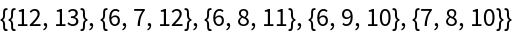### Properties and Relations

The main difference between Select and Subsets, and SelectSubsets is the amount of memory used:

 In:=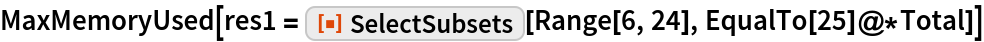Out=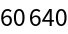Compared to naive implementation, which requires roughly 1000 times more memory:

 In:=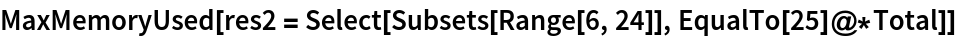Out=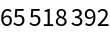Verify the result is the same:

 In:=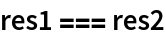Out=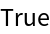With a criterion that is a tautology, SelectSubsets and Subsets give the same results:

 In:=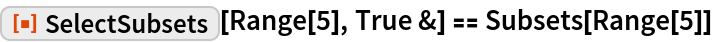Out=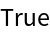### Possible Issues

SelectSubsets might not be able to return the number of elements that are requested:

 In:=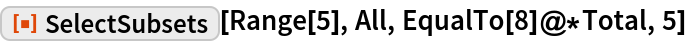Out=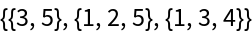### Neat Examples

Find subsets that add up to 0:

 In:=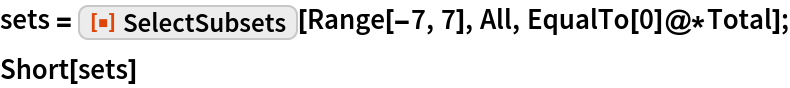Out=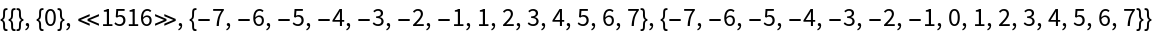Visualize the lengths of the lists:

 In:=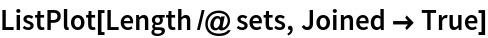Out=